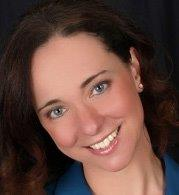All Articles

# Colloquium Speaker – Mireille Boutin Thu, Sept 29, 4:10pm, Carver 268

### Invariant Representations for Object Recognition and Symmetry DetectionIn many engineering applications, the objects of interest are either invariant under certain groups of transformations or are characterized by certain types symmetries. In this talk, we will present object representation methods that exploit such invariances or symmetries in order to be more application friendly (e.g., less computation or more robustness.)
To illustrate how invariances can be exploited in applications, we will begin with the case of an object represented by a set of points in a vector space. We will assume that the object is unchanged by a (simultaneous) rigid motion of all the points, as well as a reordering of the points. (For example, the points could represent the minutia of a fingerprint image.) In this case, we showed that any generic such object can be represented without any loss of information by the multi-set of (unordered) pairwise distances between the points. This “bag of pairwise distances” representation can also be viewed as the distribution of an invariant (the distance). More generally, invariant statistics can be used to represent complex (generic) objects without any information loss. For example, we will describe how complete weighted graphs can be represented by two invariant statistics; for a generic choice of weights, these statistics are a lossless representation of the original graph. This allows for quick comparison (polynomial time) of two generic graphs modulo isomorphism.
To illustrate how symmetries can be exploited in applications, we will look at the problem of recognizing an image representing an object with a known symmetry. We will present a new representation called the Pascal Triangle of the image, which is written in terms of complex moments of the image and has a direct connection with the radon transform. We will show how different types of symmetries manifest themselves in the Pascal triangle, and show how we applied these observation to quickly recognize HAZMAT signs (which are characterized by a 4-fold rotational symmetry) using a smart phone equipped with a camera.
We will finish the talk by briefly discussing other (current and future) signal processing and machine learning problems where geometry plays an important role.